<

## Curvature of the Earth

A cache by nognod Message this owner
Hidden : 07/14/2012
Difficulty: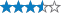Terrain: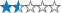Size: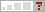(other)

#### Watch

How Geocaching WorksPlease note Use of geocaching.com services is subject to the terms and conditions in our disclaimer.

### Geocache Description:

OK ok, you can google the correct answer in a few seconds, but that’s not the point. You can actually measure it yourself as a practical experiment and log this cache.

Please message me the necessary measurements before logging the cache, but there is no need to wait for a confirmation reply before posting your log

This cache is best done on a clear day with good visibility and in the morning with the sun in the East. Useful but not essential are binoculars and a camera.

Standing at the co-ordinates of this Earthcache you have a comanding view over Brighton and the English channel. As you look at the sea beneath you, you are looking more or less sideways at the surface of a huge sphere. The sea curves away from you in every direction. The horizon line is surprisingly close, more of that later.It is possible using this horizon line and a couple of known fixed points to determine the radius of the planet using just some school-level geometry.

The location of this earthcache has been chosen very carefully. Sussex heights, the tallest building in Brighton is to the west and the roof of the top floor penthouse flat is exactly level with the horizon line of the channel.If the earth was flat, that would mean that your eye is at exactly the same altitude as the roof of Sussex house. But in fact you are looking ever so slightly down on the roof because you are higher than it. If the earth was flat the horizon would be more like here :### Geometry

The diagram below depicts this view with the curve exaggerated.Point O is your eye. Point B is on the roof of Sussex heights and point H is the furthest point of the sea that you can see on the horizon. The amount that you are looking down from the horrizontal is angle a.

Now lets zoom out to look at more than half of the planet.Point O and H are the same as before. Point C is the centre of the earth. Distance r is the radius of the earth and distance hO is the height of point O above sea level. The line from O to H is perpendicular to the line from H to C so the angle between line OC and line HC is also a. So if you know angle a and the altitude of your eye hO you can calculate r

Cos (a) = r / (r + hO)

Rearrange

r x Cos (a) + hO x Cos (a) = r
r x ( 1 – Cos(a) ) = hO x Cos (a)
r = hO x Cos (a) / (1 – Cos (a))
Call this Equation 2

So we have determined that if we know the value of the small angle a we can calculate the radius of the earth.

Lets zoom right back in to Brighton to find a way of calculating angle a.This picture also exaggerates the angle a. The line of sight to the horizon is O to B to H, same as the previous pictures. hB is the height of the roof of Sussex heights above sea level (which is 102m). Height hO is the height of your eyes above sea level. Height h is just the difference hO – hB. Distance d is the distance between your eye and sussex heights.

As the diagram shows, because line XB is parallel to the line above it, the angle OBX is also angle a. I know there is a bit of an approximation here because of course the curvature of the earth means sussex heights is tilted away from the line leading down from O, but so long as d is small compared to OH its an OK approximation.

Geometry again

Tan (a) = h / d = (hO – hB) / d
Angle a = ARC Tan { (hO – hB) / d }
Call this Equation 1

So to find angle a we need to know d, and hO and hB.

### Sussex Heights hB

If you look up the height of Sussex heights online some sites quote it as 102m and others as 82.3m above ground level. It is certainly not 102m, unless they are including the mast thingy on top of it in that figure. I have measured it at 87m above the level of the ground in Regency square using a makeshift theodolite. The ground I measured it from was 15m above sea level, so the roof is 102m above sea level. Unless you want to hang around in Regency square with a theodolite too, take it from me that hB = 102m

I’m going to leave it to the cacher to find d and hO

### Finding d

find the distance between two points of longitude and latitude

### Finding hO

Assume hO is the altitude you are now at above sea level plus 1.5m for the height of your eyes above the ground. Your GPS may give you a reading of altitude at GZ, but it is unlikely to be accurate enough to use for this calculation. Better to use the exact height mapping of the Ordnance survey.

The grid reference of the cache is TQ 32901 04558

Look around where you are standing at GZ and compare it to this map. You should be standing pretty much exactly on one of the contour lines, but which one.If you have some binoculars and it is clear enough you can use them to make sure you are at the correct height at GZ. The following photo is taken from the Too Low Waypoint a few yards down the hill at N 50 49.514 , W 000 06.876.And this is taken from the too High Waypoint a few yards up the hill at N 50 49.525 , W 000 06.850Use the map to work out which contour line you are standing on. Add 1.5m for your eye level and that’s the value of hO.

So now you have hO, hB and d you can find angle a with equation 1

Angle a = ARC Tan { (hO – hB) / d },

And then find r with equation 2

r = hO x Cos (a) / (1 – Cos (a))

If you want to be really rigorous about it then find out if the tide is in or out and adjust hO and hB for that, but it doesn’t make much difference to the result.

You should get a value for the radius of the earth in metres that is within about 20% of the correct value.

Hey presto, you have proved the earth is not flat and calculated how big it is !

Also optionally take a picture of the view, especially if you have a nice long telephoto lens

### Margin of error

When you get your answer I bet you will immediately google the correct value to see how far out you are. Bear in mind that this is a difficult measurement to make. If you are within 5% of the correct answer, that is an excellent result. Because angle a is so small, the value of h is absolutely critical. After getting your answer, try adding or subtracting a couple of meters from your value of h and see what a huge effect it has on the value of r.

### More interesting stuff to think about

Now you have the value of r you can calculate how far it is to the horizon line (lets call it z). Using the equilateral triangle OHC we get
(r x r) + (z x z) = (r + hO) x (r + hO)

Rearranging

z = sqrt { hO x ( hO + 2r ) }

This picture was taken from GZ with a long lens.In this picture you can clearly see Worthing pier which is 18km away and the white tent like structure above it of Butlins at Bognor which is 38.8km away. The land behind sussex heights is Selsey bill 45km away and very slightly behind the horizon (which is 38km away)

Find...

### 61 Logged Visits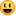55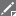5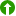1

**Warning! Spoilers may be included in the descriptions or links.Loading Cache Logs...

Current Time:
Last Updated:
Rendered From:Unknown
Coordinates are in the WGS84 datum